Test: Continuous Time Fourier Transformer (CTFT)- 2

# Test: Continuous Time Fourier Transformer (CTFT)- 2

Test Description

## 10 Questions MCQ Test GATE ECE (Electronics) 2023 Mock Test Series | Test: Continuous Time Fourier Transformer (CTFT)- 2

Test: Continuous Time Fourier Transformer (CTFT)- 2 for Electronics and Communication Engineering (ECE) 2023 is part of GATE ECE (Electronics) 2023 Mock Test Series preparation. The Test: Continuous Time Fourier Transformer (CTFT)- 2 questions and answers have been prepared according to the Electronics and Communication Engineering (ECE) exam syllabus.The Test: Continuous Time Fourier Transformer (CTFT)- 2 MCQs are made for Electronics and Communication Engineering (ECE) 2023 Exam. Find important definitions, questions, notes, meanings, examples, exercises, MCQs and online tests for Test: Continuous Time Fourier Transformer (CTFT)- 2 below.
Solutions of Test: Continuous Time Fourier Transformer (CTFT)- 2 questions in English are available as part of our GATE ECE (Electronics) 2023 Mock Test Series for Electronics and Communication Engineering (ECE) & Test: Continuous Time Fourier Transformer (CTFT)- 2 solutions in Hindi for GATE ECE (Electronics) 2023 Mock Test Series course. Download more important topics, notes, lectures and mock test series for Electronics and Communication Engineering (ECE) Exam by signing up for free. Attempt Test: Continuous Time Fourier Transformer (CTFT)- 2 | 10 questions in 30 minutes | Mock test for Electronics and Communication Engineering (ECE) preparation | Free important questions MCQ to study GATE ECE (Electronics) 2023 Mock Test Series for Electronics and Communication Engineering (ECE) Exam | Download free PDF with solutions
 1 Crore+ students have signed up on EduRev. Have you?
Test: Continuous Time Fourier Transformer (CTFT)- 2 - Question 1

### If x(n) = (1/2)n u(n), y(n) = x2(n) and (eiω) be the fourier transform of y(n), then y(e0) is

Detailed Solution for Test: Continuous Time Fourier Transformer (CTFT)- 2 - Question 1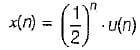and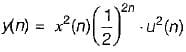⇒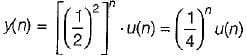then,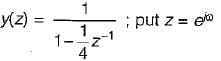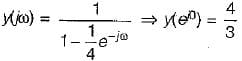Test: Continuous Time Fourier Transformer (CTFT)- 2 - Question 2

### For a signal x(t), the fourier transform is X(f), then inverse fourier transform of x(3f + 2) is given by

Detailed Solution for Test: Continuous Time Fourier Transformer (CTFT)- 2 - Question 2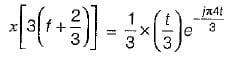Apply scaling and shifting property.

Test: Continuous Time Fourier Transformer (CTFT)- 2 - Question 3

### The Fourier transform of given signal x(t)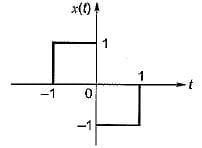Detailed Solution for Test: Continuous Time Fourier Transformer (CTFT)- 2 - Question 3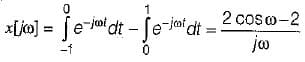Test: Continuous Time Fourier Transformer (CTFT)- 2 - Question 4

Fourier transform of x(t) = eat u (-t), a > 0 is

Test: Continuous Time Fourier Transformer (CTFT)- 2 - Question 5

Determine the fourier transform of the signal x(t) shown in figure.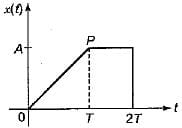Detailed Solution for Test: Continuous Time Fourier Transformer (CTFT)- 2 - Question 5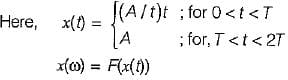⇒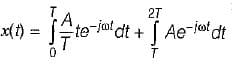⇒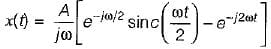Test: Continuous Time Fourier Transformer (CTFT)- 2 - Question 6

Match List-I [Function f(t)] with List-II [Fourier transform F(ω)] and select the correct answer using the codes given below the lists:
List- I
A. f(t – t0)
B. f(t) ejω0t
C. f1(t) . f2(t)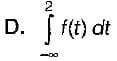List-ll
1. f(ω – ω0)
2.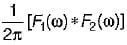3.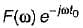4.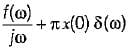5.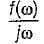Codes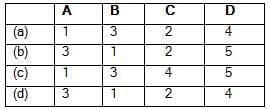Test: Continuous Time Fourier Transformer (CTFT)- 2 - Question 7

Consider a continuous time low pass filter whose impulse response h(t) is known to be real and whouse frequency response magnitude is given by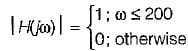Determine the value of h(t) if the group delay function is specified as t(ω) = 5.

Detailed Solution for Test: Continuous Time Fourier Transformer (CTFT)- 2 - Question 7

Since,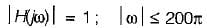0; otherwise
using duality property,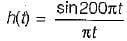Since group delay is constant and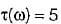So,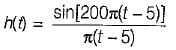Test: Continuous Time Fourier Transformer (CTFT)- 2 - Question 8

Consider a signal x1(t) having a fourier transform x1(jω). An another signal x2(t) having fourier transform x2(jω) is related to x1 (t) by x2(jω) = [1 +sgn(ω)] x1(jω) x2(t) in terms of x1(t) is equal to

Detailed Solution for Test: Continuous Time Fourier Transformer (CTFT)- 2 - Question 8

Since,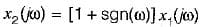or,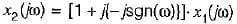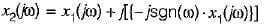or,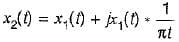because,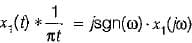using, Hilbert transform

Test: Continuous Time Fourier Transformer (CTFT)- 2 - Question 9

A Fourier transform pair is as follows: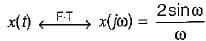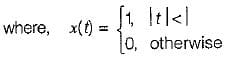The Fourier transform of given signal y(t) is

Detailed Solution for Test: Continuous Time Fourier Transformer (CTFT)- 2 - Question 9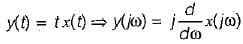⇒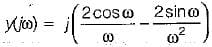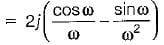Test: Continuous Time Fourier Transformer (CTFT)- 2 - Question 10

Suppose; y(t) = x(t) cost
and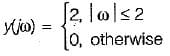then x(t) will be

## GATE ECE (Electronics) 2023 Mock Test Series

21 docs|263 tests
Information about Test: Continuous Time Fourier Transformer (CTFT)- 2 Page
In this test you can find the Exam questions for Test: Continuous Time Fourier Transformer (CTFT)- 2 solved & explained in the simplest way possible. Besides giving Questions and answers for Test: Continuous Time Fourier Transformer (CTFT)- 2, EduRev gives you an ample number of Online tests for practice

## GATE ECE (Electronics) 2023 Mock Test Series

21 docs|263 tests(Scan QR code)